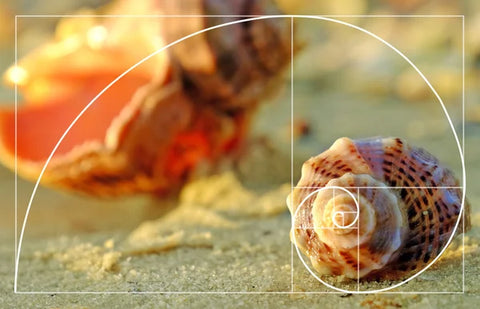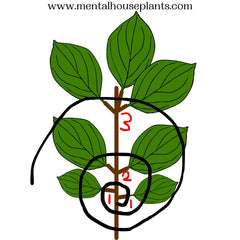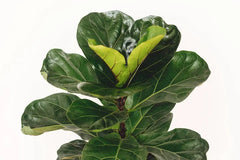# Fibonacci's Fiddle-Leaf Fig (Ficus Lyrata): The Spiraling Natural Mathematic Phenomena

## Introduction

Nature is full of hidden mathematical wonders, and one such marvel is the occurrence of the Fibonacci sequence in various elements of the natural world. From the arrangement of flower petals to the shape of seashells, this mathematical pattern reveals itself in breathtaking harmony. In this blog post, we explore the captivating relationship between the Fibonacci sequence and the growth pattern of the Ficus fiddle leaf fig plant.

## Fibonacci Sequence: A Brief Overview

To understand the significance of the Fibonacci sequence in the Ficus, let's take a moment to explore the essence of this mathematical phenomenon. The Fibonacci sequence is a series of numbers in which each number is the sum of the two preceding ones: 0, 1, 1, 2, 3, 5, 8, 13, 21, and so on. This sequence is derived from a simple rule: add the two previous numbers to obtain the next number in the sequence.The intriguing aspect of the Fibonacci sequence is its prevalence in the natural world. It appears in the spiraling patterns of pinecones, the growth of sunflower seeds, the branching of trees, and on nautilus shells (pictured to the right). Now, let's dive into how the Ficus fiddle-leaf fig showcases this mesmerizing pattern.

## The Growth Pattern of the Ficus Fiddle-Leaf FigThe Ficus Fiddle-Leaf Fig (Ficus Lyrata) is a popular houseplant known for its large, lush leaves and distinctive shape. Understanding its growth pattern helps us appreciate the connection to the Fibonacci sequence.

The Fiddle-Leaf Fig plant has broad, leathery leaves with prominent veins. The leaves are typically arranged in an alternate pattern along the stem, creating an elegant and symmetrical appearance. The plant can grow to impressive heights, with the leaves extending outward in a beautiful display.

In the Fiddle-Leaf Fig, the Fibonacci sequence manifests in the number and arrangement of its leaves along the stem. The sequence determines the distance between each leaf node, creating a spiral pattern as the plant grows. This arrangement ensures efficient exposure to sunlight and efficient use of resources.As the Fiddle-Leaf Fig grows, new leaves emerge at specific intervals along the stem. These leaf nodes follow a spiral pattern known as the Fibonacci spiral, which can be observed in the image to the left. The Fibonacci sequence dictates the angle at which each leaf emerges, resulting in a graceful curve that mimics the Golden Ratio—an aesthetically pleasing ratio found in many natural forms.

## Observing Fibonacci in Action

To identify the Fibonacci spiral in a Ficus, start by examining the arrangement of leaves along the stem. Look for a consistent pattern where new leaves emerge at specific intervals and angles. Notice how the leaves curve gently around the stem, forming a spiral shape.

To recognize the Fibonacci pattern, count the number of leaf nodes between two spirals in the fiddle-leaf fig. You'll find that the number of nodes corresponds to the Fibonacci sequence: 1, 1, 2, 3, 5, 8, 13, and so on. This consistent numerical progression reflects the inherent mathematical order found in nature.You can see in an example in my crude drawing to the right. Looking at where the spiral starts, you can see two separate leaf nodes marked 1 and 1. Moving up the Fiddle-Leaf Fig / spiral you can see a pair of leaf nodes together marked with a 2 (which is the sum of the two previous integers 1+1). Finally, the next set of leaf nodes has a total of 3 (the sum of the two previous integers 2 + 1)

## The Significance of Fibonacci's Sequence in the Ficus Fiddle-Leaf Fig

The Fibonacci spiral in the growth pattern of the Fiddle-Leaf Fig adds a remarkable aesthetic appeal to the plant. The graceful curvature and balanced arrangement of leaves create a visually captivating display that is pleasing to the eye.The Fibonacci pattern in the Fiddle-Leaf Fig's growth exemplifies the harmony and balance found in nature. It is a testament to the inherent order and mathematical precision underlying the natural world. The plant's growth reflects the perfection of the Fibonacci sequence, creating a sense of harmony in its form.

The presence of the Fibonacci sequence in the growth pattern of the Fiddle-Leaf Fig has captivated the interest and admiration of both plant enthusiasts and mathematicians. It serves as a beautiful intersection between art and science, inspiring awe and appreciation for the wonders of the natural world.

The presence of the Fibonacci sequence and spiral in the Fiddle-Leaf Fig holds more significance than just a visual marvel. It reflects the underlying mathematical principles that govern the growth and development of plants and other natural phenomena.

1. Efficient Resource Distribution: The Fibonacci spiral ensures optimal distribution of resources, such as sunlight, water, and nutrients, among the leaves of the Fiddle-Leaf Fig. Each leaf occupies a position that maximizes its access to these essential resources, allowing the plant to thrive and grow efficiently.
2. Self-Similarity and Fractal Nature: The Fibonacci spiral exhibits self-similarity and a fractal nature, meaning that the spiral pattern repeats at different scales. This property can be observed not only in the overall shape of the plant but also in the arrangement of smaller leaf clusters within each larger spiral. This self-similarity is a testament to the underlying mathematical order present in nature.
3. Aesthetics and Visual Harmony: The Fibonacci spiral in the Fiddle-Leaf Fig creates an aesthetically pleasing and visually harmonious arrangement. Our innate appreciation for these proportions may stem from the fact that the golden ratio appears frequently in art, architecture, and nature, evoking a sense of balance, beauty, and proportion.

By understanding the significance of Fibonacci's influence on the Fiddle-Leaf Fig, we gain a deeper appreciation for the intricate connections between mathematics and the natural world.

## ConclusionThe growth pattern of the Ficus Fiddle-Leaf Fig exemplifies the manifestation of the Fibonacci sequence in nature. The spiral arrangement of leaves, guided by the sequence, creates a visually stunning and mathematically precise pattern.

The presence of the Fibonacci sequence in the Ficus Fiddle-Leaf Fig's growth pattern highlights the inherent beauty and mathematical wonder found in nature. It reminds us of the intricate and harmonious design present in even the simplest aspects of the natural world.

Ready to bring the elegance of the Fibonacci spiral into your own space? Explore our exquisite Ficus collection and discover the natural beauty of these remarkable plants.